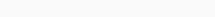# Math Puzzles Puzzle 51 Answer AndroidMath puzzle game level 51 solution with detailed hints and explanation, Scroll below to find out.

Math puzzles increase your logical thinking. The objective of the game is to find patterns hidden in the puzzle in order to arrive at the solution. Challenge yourself with different levels of math puzzles and stretch the limits of your intelligence.

### Math Puzzles Puzzle 51:

if 8809=6,7111=0

2172=0, 6666=6

11111=0, 7662=2

0000=4, 3333=0

then 2581=?Solution/Hint: Count the number of closed shapes in digits

like 0 contain 1 closed shape

6 contain 1,

8 contain 2 closed shapes

1,5,2,3,7, do not contain any closed shape

so now coming to the question, we have only two shapes in 8 so 2 is the answer.

If there is any Doubt or you are unable to understand the solution, then please let me know in comments, I will surely help you.

### 2 thoughts on “Math Puzzles Game Level 51 Answer with Solution”

•April 19, 2019 at 21:48

How many Rings/Circles Each digit contain
0 have one circle
8 have two circles (one above one below)
3 have zero
6 have One

•